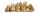# Even / odd numbers

a / Using variable n write two consecutive odd numbers.
b / the sum of three consecutive odd numbers is 333. What are this numbers?

Result

a =  109
b =  111
c =  113

#### Solution:Leave us a comment of example and its solution (i.e. if it is still somewhat unclear...):

Showing 0 comments:Be the first to comment!#### To solve this example are needed these knowledge from mathematics:

Do you have a linear equation or system of equations and looking for its solution? Or do you have quadratic equation?

## Next similar examples:

1. TwentyTwenty rabbits are put in 4 cells so that there are different number of rabbits in each cell contains at least 3 rabbits. What is the largest possible number of rabbits in one cell
2. Dropped sheetsThree consecutive sheets dropped from the book. The sum of the numbers on the pages of the dropped sheets is 273. What number has the last page of the dropped sheets?
3. Number with onesThe first digit of the number is 1, if we move this digit to the end we get a 3 times higher number, which is the number?
4. GivenGiven 2x =0.125 find the value of x
5. EquationSolve the equation: 1/2-2/8 = 1/10; Write the result as a decimal number.
6. ExpressionSolve for a specified variable: P=a+4b+3c, for a
7. Unknown number 11That number increased by three equals three times itself?
8. One-thirdA one-third of unknown number is equal to five times as great as the difference of the same unknown number and number 28. Determine the unknown number.
9. Unknown numberIdentify unknown number which 1/5 is 40 greater than one tenth of that number.
10. Negative in equation2x + 3 + 7x = – 24, what is the value of x?
11. Simple equationSolve the following simple equation: 2. (4x + 3) = 2-5. (1-x)
12. Forest nurseryIn the forest nursery after winter, they found that 1/10 stems died out of them. For them, they land 193 new spruces. How many spruces are in the forest nursery?
13. CakesGrandmother baked cakes. Half of its was poppy, quarter with plum jam and 16 cheesecakes. How many cakes she baked in total?
14. Equation?
15. Equation 29Solve next equation: 2 ( 2x + 3 ) = 8 ( 1 - x) -5 ( x -2 )
16. Simple equationSolve for x: 3(x + 2) = x - 18
17. Find xSolve: if 2(x-1)=14, then x= (solve an equation with one unknown)